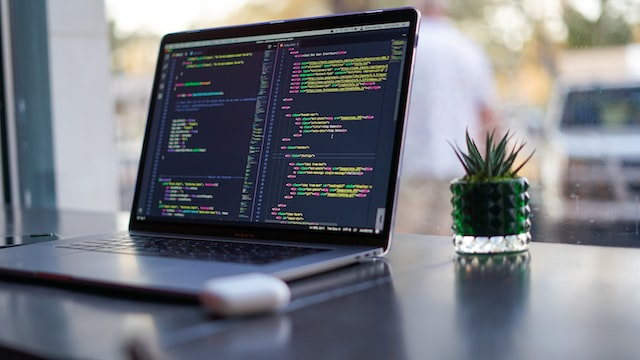# What Kinds of Math are Used in Programming

Programming is an interactive process, and at times, it can be tedious. Sometimes it can come down to an equation. With the help of math, programming becomes a more manageable task and more interactive, turning down what would have been huge research projects into a few data inputs.Image Source

In this article, we will explore the different kinds of math that are used in programming. They are mathematical operations, geometry, and calculus.

Maths students often find the subject intimidating, but it is an important subject to learn to be a successful programmer. You can make use of some math assignment help. Some math that college students need to know for programming are basic algebra, geometry, trigonometry, and calculus. Suppose you are interested in programming and would like to include mathematics in your studies. In that case, you should consider taking courses such as Calculus I and II or Statistics and Probability.

### Binary Mathematics

The field of programming relies heavily on binary mathematics, making it one of the most significant subfields of mathematics.

Binary mathematics also serves as the industry’s central focus. As a result, this subfield of mathematics is the one to focus on to become proficient in programming. To represent each number in a computer’s code, binary code, which uses the binary number system rather than the more commonplace decimal system, is utilized.

The process of coding can be made easier with the use of binary code, which is also necessary for the low-level instructions required for hardware programming. You will need to be familiar with the hexadecimal numbering system to do certain actions, such as changing the color of an item. In addition to this, the traditional arithmetic operations of addition, subtraction, multiplication, and division are used inside the realm of binary mathematics.

### Algebra

Students need to be proficient in college algebra to move on to more advanced levels of mathematics because it is one of the foundational mathematics subjects. Beyond the most fundamental arithmetical operations, algebra is one of the most established branches of mathematical study.

The emphasis is placed on graphs and equations, as well as the ability to solve equations with variables that are not known. Programming computers requires an understanding of algebra to create algorithms and software that can operate with mathematical functions. In addition to this, it participates in the design of programs for numerical programs.

### Statistics

A branch of mathematics known as statistics education concludes data sets by employing quantified models, representations, and summaries. The discipline of computer science is similar to many others in that statistical analysis can serve a wide variety of functions, which is also true in many other fields.

Researchers can deduce conclusions about underlying trends and make predictions about future behavior and trends through the use of statistics, which allows for the analysis of data. The analysis of regression, the mean, variance, analysis of variance, skewness, and kurtosis are all examples of metrics that are utilized in statistics.

The field of computer science employs statistics in the process of regression analysis, as well as in data mining, information extraction, traffic modeling, voice recognition, sense of direction and image processing, and artificial intelligence. Statistics is also used in the study of images.

### Calculus

Calculus is the study of change through time by calculating the derivatives and integrals of functions. Calculus, as a consequence of this, is often split into two subfields that are complementary to one another: differential calculus and integral calculus.

The study of rates of change when a quantity is known is the focus of differential calculus. Integral calculus, on the other hand, focuses on studying quantities even when a rate of change is already known.

Calculus is useful in computer science, just as in other disciplines, particularly when working with problems involving rates of change. Calculus is utilized in the field of computer science for the creation of graphs and other visualizations, the programming of applications, the development of ways to answer statistical questions, applications that facilitate problem-solving, simulations, and the design and analysis of algorithms.

### Discrete Mathematics

Discrete mathematics is a prerequisite for any computer science degree because it is the heavyweight of the computer science math fields. When we talk about discrete mathematics, we’re talking about things that can be represented in a finite number of ways utilizing techniques such as logic, numbers, graphs, and probability. Discrete mathematics is crucial to all aspects of computer science.

### Conclusion

The kind of math you have to master all depends on the type of programming language or framework you are interested in.

Different programming languages or frameworks require different levels of knowledge, so it is crucial to know what type of math you need before starting the class and enrolling in the course.

This site uses Akismet to reduce spam. Learn how your comment data is processed.